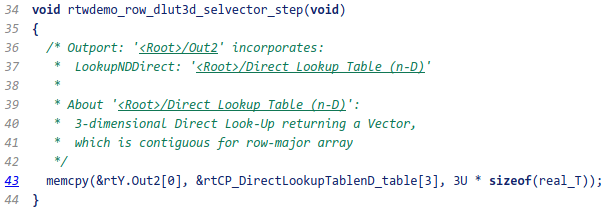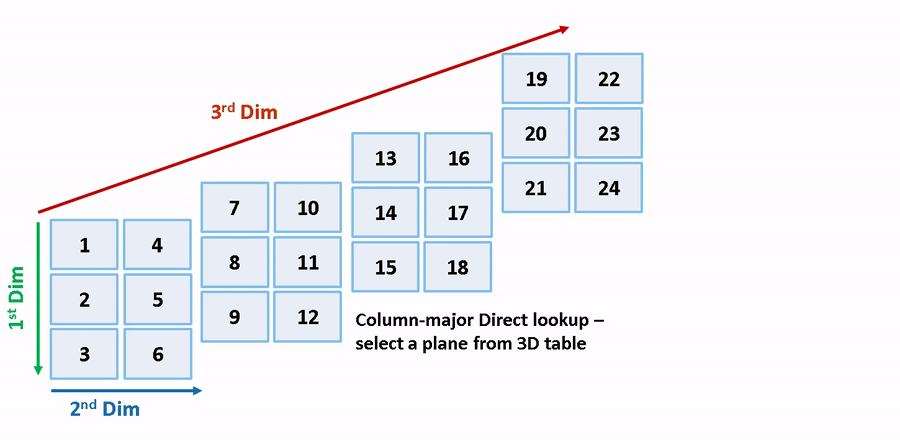# Direct Lookup Table Algorithm for Row-Major Array Layout

This example shows how Simulink selects a vector or a 2-D matrix from table data. In a 2-D table, the output vector can be a column or a row depending on the model configuration setting Use algorithms optimized for row-major array layout. In this example, the Direct Lookup Table algorithm is optimized for row-major array layout. The Direct Lookup Table algorithm that is optimized for column-major array layout is also presented as a reference. The code generated by using row-major interpolation algorithm performs with the best speed and memory usage when operating on table data with row-major array layout. The code generated by using column-major algorithm performs best with column-major array layout.

In this example, you:

• Output a vector or a plane by using direct lookup with a column-major or a row-major algorithm.

• Preserve semantics when switching from a column-major algorithm to a row-major algorithm.

• Generate code by using a row-major algorithm and an array layout.

### Simulate by Using Row-Major Algorithm — Output a Vector from 3-D Table

Open example models `rtwdemo_row_dlut3d_selvector` and `rtwdemo_col_dlut3d_selvector`.

``` open_system('rtwdemo_row_dlut3d_selvector'); open_system('rtwdemo_col_dlut3d_selvector'); ```1. By default, Simulink configures a model with column-major algorithm and column-major array layout. The model `rtwdemo_col_dlut3d_selvector` is preconfigured to use column-major algorithms. Simulate the model and observe the output stored in workspace variable `yout`.

2. To enable row-major algorithms, open the Configuration Parameters dialog box. On the Math and Data Types pane, select the configuration parameter Use algorithms optimized for row-major array layout (Simulink) Alternatively, in the MATLAB Command Window, enter:

```set_param('rtwdemo_col_dlut3d_selvector','UseRowMajorAlgorithm','on'); ```

3. On the Simulation tab, click Run to simulate the model. Observe the change in output dimension and numeric values logged in workspace variable `yout`.

The column-major and row-major algorithms differ semantically in the selection of output vector. For example, in a 2-D table, Simulink selects a column vector as output for column-major algorithm and a row vector for row-major algorithm. In a table with a 3-D or higher dimension, Simulink selects the output vector from the first dimension of the table for a column-major algorithm and from the last dimension of the table for a row-major algorithm. The elements of the selected vector are contiguous in the table storage memory. In this example, the last dimension is the third dimension of the 3-D table. Due to semantic change, column-major and row-major direct lookup table algorithms output different vector size and numeric values.

These illustrations compare the vector output of row-major and column-major direct lookup table algorithms in a 3-D table.### Preserve Semantics by Using Table Permutation

For a direct lookup table that outputs a vector or 2-D matrix, the model semantics change when you switch from a column-major algorithm to a row-major algorithm. To preserve the semantics or ensure the same output given the same block I/O connections, you must permute the table data. Otherwise, Simulink propagates incorrect dimensions to downstream blocks.

1. The block `rtwdemo_col_dlut3d_selvector/Direct Lookup Table (n-D)` has 3-D table data T3d = reshape([1:24], 3,2,4) and two input ports with value 0 and 1 (both are 0-based indices). The selected output vector is T3d(:,1,2) (1-based index) for a column-major algorithm. To preserve the semantics for a row-major algorithm on the same model, that is, select the same vector with same index port inputs, permute the table as T3d_p = permute(T3d, [2,3,1]). For a row-major algorithm, the selected vector is T3d_p(1,2,:).

```T3d_str = get_param('rtwdemo_col_dlut3d_selvector/Direct Lookup Table (n-D)','Table'); set_param('rtwdemo_col_dlut3d_selvector/Direct Lookup Table (n-D)','Table',... ['permute(',T3d_str,',[2,3,1])']); ```

2. When you import table data from a file, you must permute the table data in the file before importing it. This permutation keeps the table tunable throughout the simulation and code generation workflow.

### Code Generation by Using Row-Major Algorithm and Array Layout

After permuting the table data, Simulink configures the model `rtwdemo_col_dlut3d_selvector` for row-major simulation. The model is equivalent to the preconfigured model `rtwdemo_row_dlut3d_selvector` that has permuted table data and uses a row-major algorithm.

1. To set up these models for row-major code generation, open the Configuration Parameters dialog box. In addition to enabling the Use algorithms optimized for row-major array layout configuration parameter, on the Code Generation > Interface pane, set the configuration parameter Array layout to the `Row-Major` option. This configuration parameter enables the model for row-major code generation. Alternatively, in the MATLAB Command Window, enter:

```% For model 'rtwdemo_col_dlut3d_selvector' set_param('rtwdemo_col_dlut3d_selvector', 'ArrayLayout','Row-major'); % For model 'rtwdemo_row_dlut3d_selvector' set_param('rtwdemo_row_dlut3d_selvector', 'ArrayLayout','Row-major'); ```

2. In the Direct Lookup Table (n-D) block dialog box, examine the permuted 3-D table data.3. Change your current folder in MATLAB® to a writable folder. On the C Code tab, click Build to generate C code. In the generated code, the `memcpy` function replaces the `for` loops. Using `memcpy` reduces the amount of memory for storing data. This optimization improves execution speed.### Simulate by Using Row-Major Algorithm — Output a Plane from 3-D Table

```open_system('rtwdemo_row_dlut3d_selplane'); open_system('rtwdemo_col_dlut3d_selplane'); ```1. Open the example model `rtwdemo_row_dlut3d_selplane` that outputs a plane or 2-D matrix from a 3-D table.

2. Simulate and generate code from the model by repeating the steps performed on `rtwdemo_col_dlut3d_selvector`. The row-major and column-major direct lookup algorithms that output a 2-D matrix from a 3-D table are illustrated here.```close_system('rtwdemo_row_dlut3d_selvector',0); close_system('rtwdemo_col_dlut3d_selvector',0); close_system('rtwdemo_row_dlut3d_selplane',0); close_system('rtwdemo_col_dlut3d_selplane',0); ```